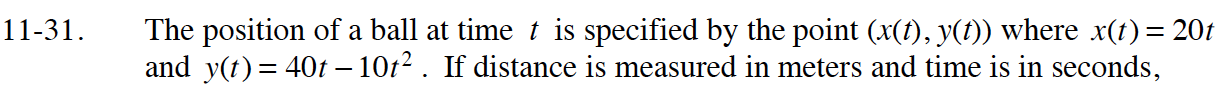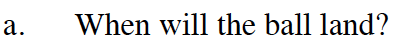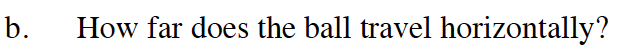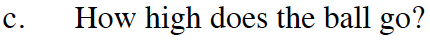### Home > CALC > Chapter 11 > Lesson 11.1.3 > Problem11-31

11-31.
1. The position of a ball at time t is specified by the point (x(t), y(t)) where x(t) = 20t and y(t) = 40t – 10t2 . If distance is measured in meters and time is in seconds, Homework Help ✎

1. When will the ball land?

2. How far does the ball travel horizontally?

3. How high does the ball go?This occurs when y(t) = 0.